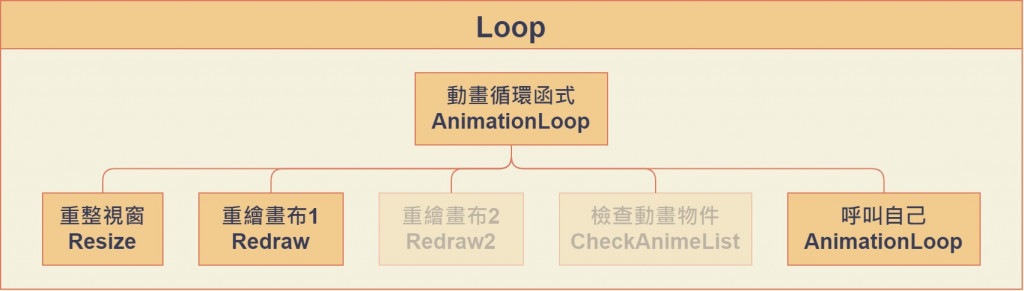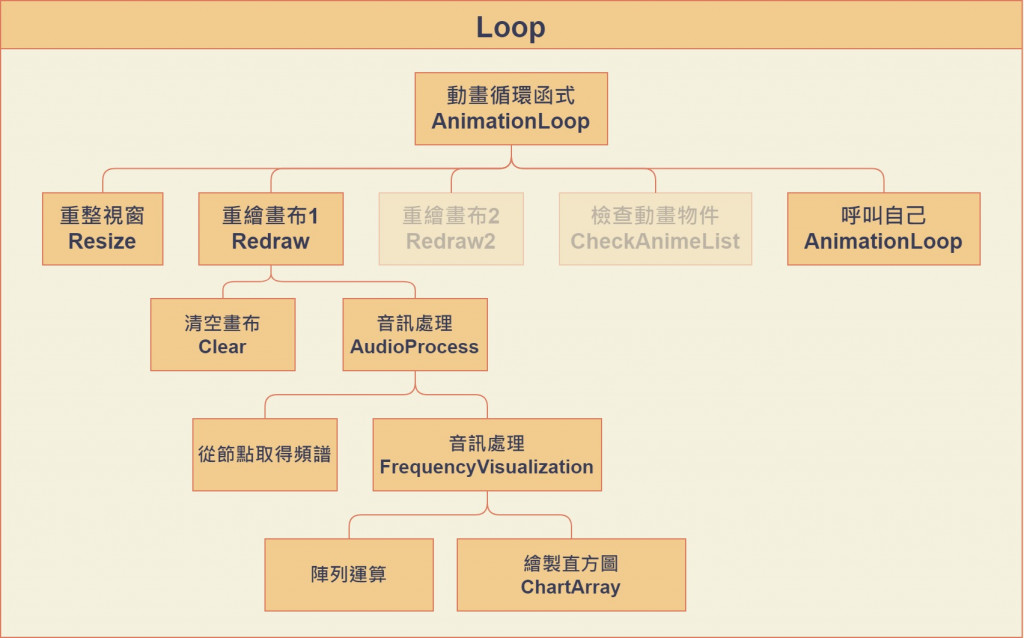DAY 5
0
Modern Web

話不多說先上圖function AnimationLoop(){
Resize("#game-box", canvas, context, '#000');
Redraw();
requestAnimationFrame(AnimationLoop);
}

Resize 重整視窗

let canvas = document.getElementById("canvas");
let context = canvas.getContext("2d");
const RATIO = 2;
let WIDTH, HEIGHT;

function Resize(boxID, canvas, context, fillStyle=undefined){
if(WIDTH != window.innerWidth * RATIO || HEIGHT != window.innerHeight * RATIO){
WIDTH = window.innerWidth * RATIO;
HEIGHT = window.innerHeight * RATIO;
let box = document.querySelector(boxID);
canvas.width = WIDTH;
canvas.height = HEIGHT;
canvas.style.width = WIDTH/RATIO + "px";
canvas.style.height = HEIGHT/RATIO + "px";
box.style.width = WIDTH/RATIO + "px";
box.style.height = HEIGHT/RATIO + "px";
if(fillStyle != undefined){
context.beginPath();
context.rect(0, 0, WIDTH, HEIGHT);
context.fillStyle = fillStyle;
context.fill();
}
}
}

Redraw 重繪畫布

function Redraw(){
clear(context);
AudioProcess();
}

1. 清空畫布
2. 從節點取得頻譜
3. 陣列運算
4. 繪製直方圖
（分別包成了不同的function放在不同位置）Clear 清空畫布

function clear(context){
// context.clearRect(0, 0, WIDTH, HEIGHT);
context.beginPath();
context.rect(0, 0, canvas.width, canvas.height);
context.fillStyle = 'rgba(0, 0, 0, 0.5)';
context.fill();
}

context.globalAlpha

AudioProcess 處理音訊

Hooray~~終於銜接至上回的音訊處理拉！希望大家都還記得我們計算頻寬的方法，忘記的罰你回去看！

function AudioProcess(){
let bands = audioCtx.sampleRate / analyserNode.fftSize * 2; // 每個區段的頻寬
let HighestBands = 16000; // 16kHz高音頻以下的音樂
let index = HighestBands / bands;
bufferLength = analyserNode.frequencyBinCount;
if(bufferLength != undefined){
dataArray = new Uint8Array(bufferLength);
analyserNode.getByteFrequencyData(dataArray);
FrequencyVisualization(dataArray, index, window.shrink);
}
else FrequencyVisualization(new Array(256).fill(0), index, window.shrink);
}

function FrequencyVisualization(dataArray, index, shrink){
const INDEX = index - index%shrink;
ChartArray(context, ReArray(dataArray), WIDTH*0.05, WIDTH*0.9, HEIGHT*0.6, HEIGHT*0.2);
function ReArray(array){
let newArray = new Array();
for (let N = 0; N <= INDEX; N = N + shrink) {
newArray[N] = 0;
for (let n = 0; n < shrink; n++) {
newArray[N + 0] = newArray[N + 0] + array[N + n] / shrink;
}
}
return newArray;
}
function ChartArray(context, array, left, right, middle, height=255){
const WIDTH = (right - left) / INDEX;
const THICK = window.thick;
context.fillStyle = Background.Transform(1.5);
context.strokeStyle = Background.Transform(1.5);
for (let N = 0 ; N <= INDEX; N = N + shrink) {
context.fillRect(left + (N) * WIDTH, middle,
PADDING * WIDTH, -(THICK + array[N] / 255 * height));
context.strokeRect(left + (N) * WIDTH, middle,
PADDING * WIDTH,   THICK + array[N] / 255 * height);
}
}
}

• window.shrink 陣列縮減的比率(相當於每個直方圖的間隔)